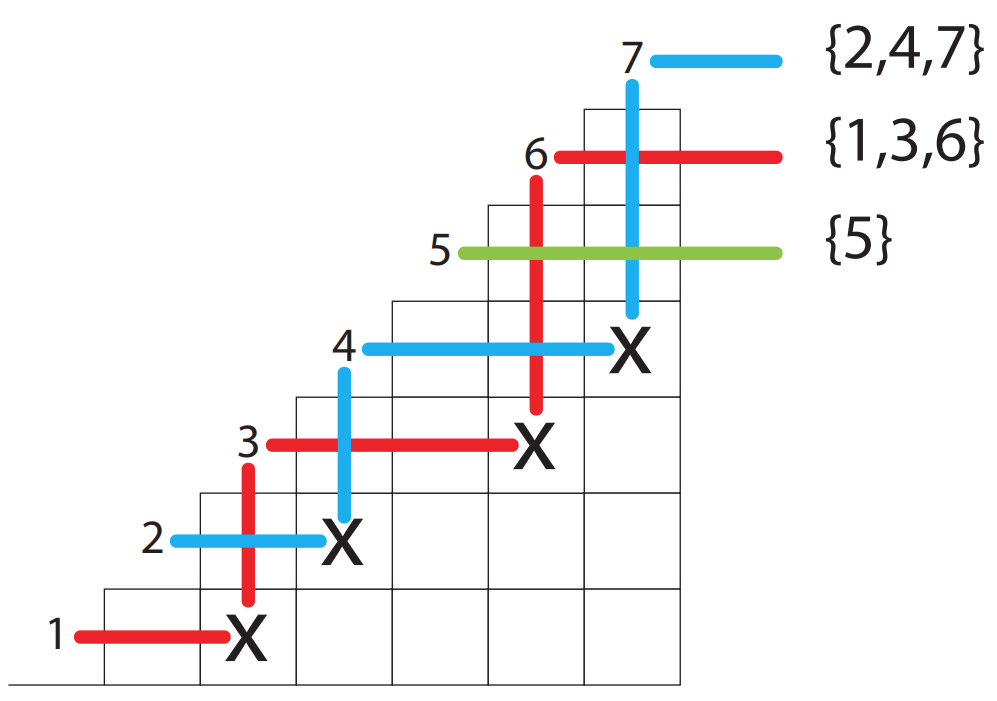## § Set partitions

Let $X$ be a set. A breakup of $X$ into pairwise disjoint sets $A[i]$ such that $\cup_i A[i] = X$ is called a partition $P$ of the set $X$.

#### § Stirling numbers of the second kind: $S(n, k)$

These count the number of ways to break an $n$ element set into $k$ partitions/equivalence classes. The recurrence is:
$S(n, k) \equiv S(n-1, k-1) + kS(n-1, k)$
• For the $n$th element, I either build a new equivalence class $\{ n \}$and then make $k-1$ equivalence classes from $\{1\dots (n-1)\}$.
• Alternatively, I have $k$ equivalence classes from $\{1 \dots (n-1)\}$, say $P, P, \dots, P[k]$I decide into which $P[i]$ the $n$ should go, which gives me $k$ choices.
• Initial conditions: $S(0, 0) = 1$, $S(0, k \neq 0) = S(n \neq 0, 0) = 0$.

#### § Stirling numbers and surjections

Interesting interpretation: The number of ways to surject an $n$ element set into a $k$ element set, since a surjection breaks a set up into a known number of fibers (in this case, $k$ fibers).
This is not entirely true, because we only get $k$ equivalence classes of the set $\{1, \dots, n\}$. We need to decide where to map each equivalence class. So the correct count of $\{ [n] \xrightarrow{onto} [k] \}$ is $k!S(n, k)$: there are $k!$ ways to map equivalence classes of $n$ to elements of $k$

#### § Rook theory(!)

Turns out we can provide a crazy relationship betweeen ferrers diagrams, and rooks (as in the chess piece) and stirling numbers of the second kind. We define $\Delta(n)$ to be the board consisting of the integer partition $[n-1, n-2, \dots, 1]$. For example, we think of $\Delta(4)$ as:
Delta(4):
+--+
|  |
+--+--+
|  |  |
+--+--+--+
|  |  |  |
+--+--+--+--+

Hopefully, this looks like a staircase with 4 stairs starting from the ground. We have filled in squares of $[3, 2, 1]$ blocks stacked above one another. We define $r(n, k)$ to be the number of legal rook placements on a board $\Delta(n)$ with $k$ free rows. That is, we have $(n-k)$ rooks to place on the board $\Delta(n)$, with one on each row, such that no rook attacks another rook.
• Boundary condition: $r(0, 0) = 0$ 0 free rows on a $\Delta(0)$ board counts as one configuration.
• Recurrence: $r(n, k) \equiv r(n-1, k-1) + k r(n-1, k)$
• $r(n-1, k-1)$ term: We don't place a rook on the bottom row. This means we have used up a free row, and need to place rooks with $(k-1)$ free rows on an $(n-1)$ board:
+--+
|  |
+--+--+
|  |  |  r(n-1, k-1)
+--+--+--+

+--+--+--+
|  |  |  |  BLANK
+--+--+--+--+

• $k r(n-1, k)$: We fill out $\Delta(n-1)$ with rooks such that we have $k$ free rows. Then, we add the final row. Note that since we have rooks, $k$ free rows is equivalent to $k$ free columns! Now, we can't leave the final row free, since we have already exhausted our $k$ free rows in the recursion. We have $k$free columns for the rook in the final row to inhabit. So we get $k r(n-1, k)$.

#### § Bijection between rooks and Stirling numbers of the second kind

Finally, note that $S(n, k) = r(n-k, k)$, as $S(n, k) = S(n-1, k-1) + k S(n-1, k)$ which is equivalent to asking:
\begin{aligned} &S(n, k) = S(n-1, k-1) + k S(n-1, k) \\ &r(n-k, k) =_? r(n-1 - (k-1), k-1) + k r(n-1 - k, k) \\ &r(n-k, k) =_? r(n-k, k-1) + k r(n-k-1, k) \\ &\text{set m = n-k: } \\ &r(m, k) = r(m, k-1) + k r(m, k) \\ \end{aligned}

#### § Directly reading off the bijection between set partitions and rook placements

I found this very cool. The idea is to treat each rook as a "bouncer" that bounces light rays. All elements hit by a light ray belong to an equivalence class.#### § Wrooks and signless stirling numbers

Similar to the rooks, we define a wrook (a weak rook) as one that only attacks on its row. Here $w(n, k)$ denotes a placement of wrooks on $\Delta(n)$ with $k$ free rows.
\begin{aligned} &w(n, k) \equiv \\ &~ w(n-1, k-1)~ \text{Leave bottom row free: uses up a free row} + \\ &~n w(n-1, k)~ \text{Place a wrook on bottom row: n possible positions} \end{aligned}
The corresponding "counting" object is called as the signless stirling numbers: TODO